7 篇文章 0 订阅

# 1.pagerank介绍

1. pagerank的简单介绍及实现，这个链接中介绍了最原始的pagerank的思路，写得也比较清楚，之后给出了实现的代码，代码写得也是非常清晰易懂，但是这个实现的pagerank是最原始的pagerank，会出现较多的问题，其迭代公式为：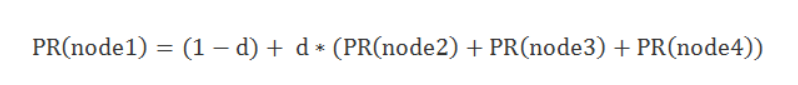2. pagerank更深入的介绍，这个链接中的介绍相对而言就更加深入了，给出的迭代公式也是经过改进之后的，这个也是网上绝大多数资料所说的pagerank。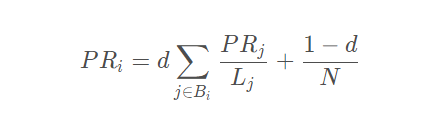3. networkx实现pagerank的源码解析官方文档，以上是一个源码解析和官方文档，networkx中的pagerank和1,2链接中的pagerank的迭代公式是不同的，源码解析中的说明也是正确的，但是我觉得写得还是不那么好理解，所以我希望进一步解释下，这也就是写本博客的缘由。并且我也通过两个例子证实第二个2链接中的迭代公式与源码解析中的公式是不一样的，但是有意思的是结果似乎是相同的，但是没有从理论上想出来是为什么，主要是数学功底不够，要是有大佬知道可以留言。

# 2.networkx实现的pagerank的说明

def pagerank(G, alpha=0.85, personalization=None,
max_iter=100, tol=1.0e-6, nstart=None, weight='weight',
dangling=None):
"""Return the PageRank of the nodes in the graph.

PageRank computes a ranking of the nodes in the graph G based on
the structure of the incoming links. It was originally designed as
an algorithm to rank web pages.

Parameters
----------
G : graph
A NetworkX graph.  Undirected graphs will be converted to a directed
graph with two directed edges for each undirected edge.

alpha : float, optional
Damping parameter for PageRank, default=0.85.

personalization: dict, optional
The "personalization vector" consisting of a dictionary with a
key for every graph node and nonzero personalization value for each node.
By default, a uniform distribution is used.

max_iter : integer, optional
Maximum number of iterations in power method eigenvalue solver.

tol : float, optional
Error tolerance used to check convergence in power method solver.

nstart : dictionary, optional
Starting value of PageRank iteration for each node.

weight : key, optional
Edge data key to use as weight.  If None weights are set to 1.

dangling: dict, optional
The outedges to be assigned to any "dangling" nodes, i.e., nodes without
any outedges. The dict key is the node the outedge points to and the dict
value is the weight of that outedge. By default, dangling nodes are given
outedges according to the personalization vector (uniform if not
specified). This must be selected to result in an irreducible transition
matrix (see notes under google_matrix). It may be common to have the
dangling dict to be the same as the personalization dict.

Returns
-------
pagerank : dictionary
Dictionary of nodes with PageRank as value

Examples
--------
>>> G = nx.DiGraph(nx.path_graph(4))
>>> pr = nx.pagerank(G, alpha=0.9)

Notes
-----
The eigenvector calculation is done by the power iteration method
and has no guarantee of convergence.  The iteration will stop
after max_iter iterations or an error tolerance of
number_of_nodes(G)*tol has been reached.

The PageRank algorithm was designed for directed graphs but this
algorithm does not check if the input graph is directed and will
execute on undirected graphs by converting each edge in the
directed graph to two edges.

--------

References
----------
..  A. Langville and C. Meyer,
"A survey of eigenvector methods of web information retrieval."
http://citeseer.ist.psu.edu/713792.html
..  Page, Lawrence; Brin, Sergey; Motwani, Rajeev and Winograd, Terry,
The PageRank citation ranking: Bringing order to the Web. 1999
http://dbpubs.stanford.edu:8090/pub/showDoc.Fulltext?lang=en&doc=1999-66&format=pdf
"""


PR=alpha * (A * PR+dangling分配)+(1-alpha) * 平均分配

dangling node 也就是悬空结点，它的出度为0，也就是无法从它到任何其他结点，解决办法是增加一定的随机性，dangling分配其实就是加上一个随机向量，也就是无法从这个结点去往任何其他结点，但是可能会随机重新去一个结点，也可以这么理解，到了一个网站，这个网站不连接到任何网站，但是浏览者可能重新随机打开一个页面。

1，A*PR这种是自由分配，大家都愿意参与竞争交流的分配

2，dangling是强制分配，有点类似打倒土豪分田地的感觉，你不参与自由市场，那好，我们就特地帮你强制分。

3，平均分配，其实就是有个机会大家实现共产主义了，不让spider trap这种产生rank sink的节点捞太多油水，其实客观上也是在帮dangling分配。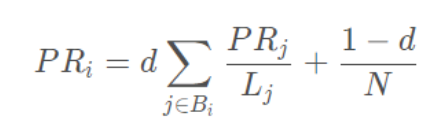networkx中的迭代公式里多了一个dangling分配，其他都是一模一样的。这个一开始我有一些难以理解，因为感觉dangling分配似乎并不是必要的，如果为了解决dangling node的问题，最后加一个随机分配就可以了，随机分配就意味着一定几率会随机跳转到一个全新的网页。接下来，我们来看一下源码中的这一部分的关键代码，来更进一步看看pr值究竟是咋样更新迭代的。

for _ in range(max_iter):
xlast = x
x = dict.fromkeys(xlast.keys(), 0)  #x初值
danglesum = alpha * sum(xlast[n] for n in dangling_nodes) #第2部分：计算dangling_nodes的PR总值
for n in x:
for nbr in W[n]:
x[nbr] += alpha * xlast[n] * W[n][nbr][weight]    #第1部分:将节点n的PR资源分配给各个节点，循环之
for n in x:
x[n] += danglesum * dangling_weights[n] + (1.0 - alpha) * p[n]   #第3部分：节点n加上dangling nodes和均分的值

# 迭代检查
err = sum([abs(x[n] - xlast[n]) for n in x])
if err < N*tol:
return x


# 3.验证说法的正确性及2个例子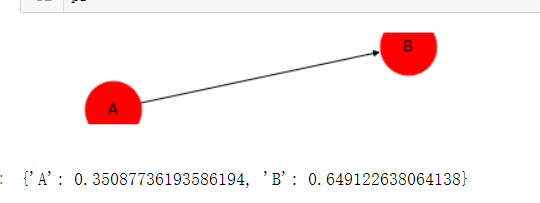P R ( A ) = a l p h a ∗ ( A ∗ P R + d a n g l i n g 分 配 ) + ( 1 − a l p h a ) ∗ 平 均 分 配 = 0.85 ∗ 0.5 ∗ 0.649123 + 0.15 / 2 = 0.350877 PR(A)=alpha * (A * PR+dangling分配)+(1-alpha) * 平均分配=0.85 * 0.5 * 0.649123 + 0.15/2 = 0.350877
P R ( B ) = a l p h a ∗ ( A ∗ P R + d a n g l i n g 分 配 ) + ( 1 − a l p h a ) ∗ 平 均 分 配 = 0.350877 + 0.85 ∗ 0.35087 = 0.649123 PR(B)=alpha * (A * PR+dangling分配)+(1-alpha) * 平均分配=0.350877+0.85 * 0.35087=0.649123P R ( A ) = 0.15 / 2 = 0.075 PR(A)= 0.15/2 = 0.075
P R ( B ) = 0.15 / 2 + 0.85 ∗ 0.075 = 0.13875 PR(B)= 0.15/2+0.85*0.075 = 0.13875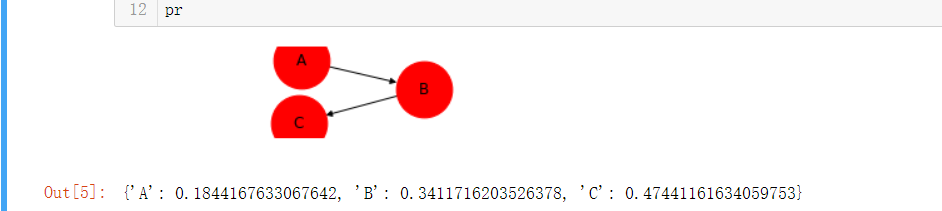# 4.猜测与结论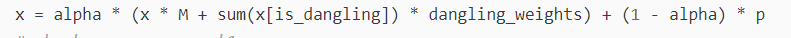05-15
10-27809110-231287
12-17836
07-2675
06-01268
12-25671
08-12761
12-279807
07-075416
09-051383
07-061万+
12-31563
09-211946
09-141061
05-181万+
11-261501
09-111164
05-23336点击重新获取扫码支付余额充值